# COUPLED INDUCTORSClick or Tap the Example circuits below to invoke TINACloud and select the Interactive DC mode to Analyze them Online.
Get a low cost access to TINACloud to edit the examples or create your own circuitsNODE POTENTIAL AND MESH CURRENT METHOD IN AC CIRCUITS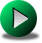PERIODIC WAVEFORMS

Two inductors or coils that are linked by electromagnetic induction are said to be coupled inductors. When an alternating current flows through one coil, the coil sets up a magnetic field which is coupled to the second coil and induces a voltage in that coil. The phenomenon of one inductor inducing a voltage in another inductor is known as mutual inductance.

Coupled coils can be used as a basic model for transformers, an important part of power distribution systems and electronic circuits. Transformers are used for changing alternating voltages, currents, and impedances, and to isolate one part of a circuit from another.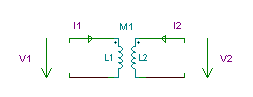Three parameters are required to characterize a pair of coupled inductors: two self inductances, L1 and L2, and the mutual inductance, L12 = M. The symbol for coupled inductors is:

Circuits which contain coupled inductors are more complicated than other circuits because we can only express the voltage of the coils in terms of their currents. The following equations are valid for the circuit above with the dot locations and reference directions shown: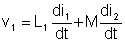Using impedances instead: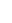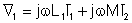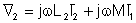The mutual inductance terms can have a negative sign if the dots have different positions. The governing rule is that the induced voltage on a coupled coil has the same direction relative to its dot as the inducing current has to its own dot on the coupled counterpart.

The T - equivalent circuit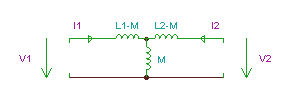is very useful when solving circuits with coupled coils.

Writing the equations you can easily check the equivalence.

Let us illustrate this through some examples.

# Example 1

Find the amplitude and initial phase angle of the current.

vs (t) = 1cos (w├Śt ) V w=1kHz

The equations: VS = I1*j w L1 - I*j w M

0 = I*j w L2 - I1*j w M

Hence: I1 = I*L2/M; and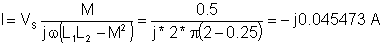i(t) = 0.045473 cos (w├Śt - 90┬░) A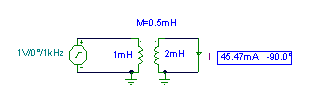Click/tap the circuit above to analyze on-line or click this link to Save under Windows

 {Solution by TINA's Interpreter} om:=2*pi*1000; Sys I1,I 1=I1*j*om*0.001-I*j*om*0.0005 0=I*j*om*0.002-I1*j*om*0.0005 end; abs(I)=[45.4728m] radtodeg(arc(I))=[-90]

# Example 2

Find the equivalent impedance of the two-pole at 2 MHz!

Click/tap the circuit above to analyze on-line or click this link to Save under Windows

First we show the solution obtained by solving the loop equations. We suppose that the impedance meter current is 1 A so that the meter voltage equals the impedance. You can see the solution in TINA's Interpreter.

 {Solution by TINA's Interpreter} {Use loop equations} L1:=0.0001; L2:=0.00001; M:=0.00002; om:=2*pi*2000000; Sys Vs,J1,J2,J3 J1*(R1+j*om*L1)+J2*j*om*M-Vs=0 J1+J3=1 J2*(R2+j*om*L2)+J1*om*j*M-J3*R2=0 J3*(R2+1/j/om/C)-J2*R2-Vs=0 end; Z:=Vs; Z=[1.2996k-1.1423k*j]

We could also solve this problem using the T-equivalent of the transformer in TINA:

Click/tap the circuit above to analyze on-line or click this link to Save under Windows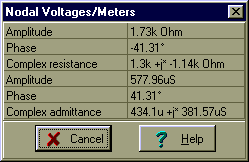If we wanted to calculate the equivalent impedance by hand, we'd need to use wye to delta conversion. While this is feasible here, in general circuits can be very complicated, and it is more convenient to use the equations for coupled coils.NODE POTENTIAL AND MESH CURRENT METHOD IN AC CIRCUITSPERIODIC WAVEFORMS